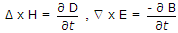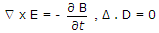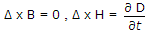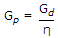# Electronics and Communication Engineering - Electromagnetic Field Theory

1.
Whenever a wave is incident on a perfect conductor then the reflection coefficient is
1
0
1 < 180°
depend upon η1, η2
Explanation:
No answer description is available. Let's discuss.

2.
Which one set of the following Maxwell's equation gives the basic idea of radiation ?Δ . D = r, Δ . B = 0Explanation:
No answer description is available. Let's discuss.

3.
When a wave is incident from the more dense into a less dense medium at an angle equal to or exceeding the critical angle, the wave suffers total internal __________ .
reflection
refraction
transmission
none of the above
Explanation:
No answer description is available. Let's discuss.

4.
Power gain and directivity related with, Gp power gain, Gd directive gain
Gp = η Gd
Gd = η GpGp = η Gd2